# Clear sky¶

This section reviews the clear sky modeling capabilities of pvlib-python.

pvlib-python supports two ways to generate clear sky irradiance:

1. A Location object’s get_clearsky() method.

2. The functions contained in the clearsky module, including ineichen() and simplified_solis().

Users that work with simple time series data may prefer to use get_clearsky(), while users that want finer control, more explicit code, or work with multidimensional data may prefer to use the basic functions in the clearsky module.

The Location subsection demonstrates the easiest way to obtain a time series of clear sky data for a location. The Ineichen and Perez and Simplified Solis subsections detail the clear sky algorithms and input data. The Detect Clearsky subsection demonstrates the use of the clear sky detection algorithm.

We’ll need these imports for the examples below.

In : import os

In : import itertools

In : import matplotlib.pyplot as plt

In : import pandas as pd

In : import pvlib

In : from pvlib import clearsky, atmosphere, solarposition

In : from pvlib.location import Location

In : from pvlib.iotools import read_tmy3


## Location¶

The easiest way to obtain a time series of clear sky irradiance is to use a Location object’s get_clearsky() method. The get_clearsky() method does the dirty work of calculating solar position, extraterrestrial irradiance, airmass, and atmospheric pressure, as appropriate, leaving the user to only specify the most important parameters: time and atmospheric attenuation. The time input must be a pandas.DatetimeIndex, while the atmospheric attenuation inputs may be constants or arrays. The get_clearsky() method always returns a pandas.DataFrame.

In : tus = Location(32.2, -111, 'US/Arizona', 700, 'Tucson')

In : times = pd.date_range(start='2016-07-01', end='2016-07-04', freq='1min', tz=tus.tz)

In : cs = tus.get_clearsky(times)  # ineichen with climatology table by default

In : cs.plot();

In : plt.ylabel('Irradiance $W/m^2$');

In : plt.title('Ineichen, climatological turbidity');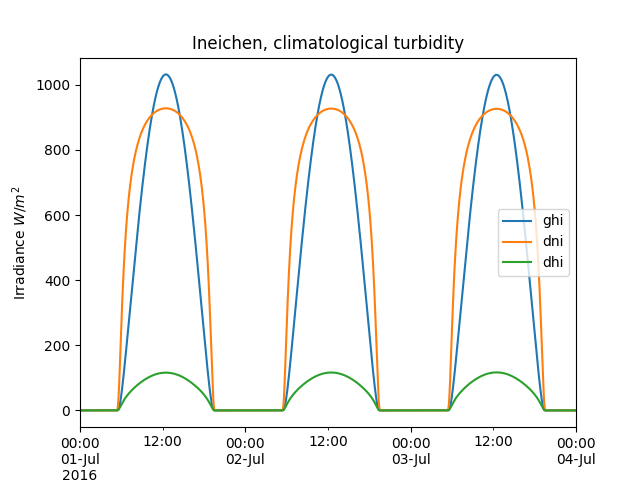The get_clearsky() method accepts a model keyword argument and propagates additional arguments to the functions that do the computation.

In : cs = tus.get_clearsky(times, model='ineichen', linke_turbidity=3)

In : cs.plot();

In : plt.ylabel('Irradiance $W/m^2$');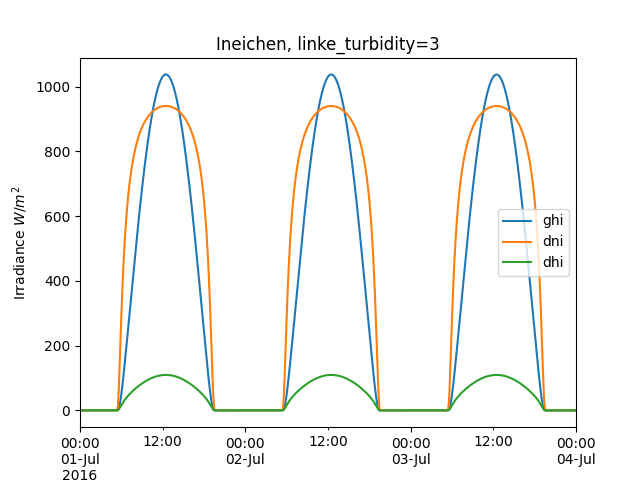In : cs = tus.get_clearsky(times, model='simplified_solis', aod700=0.2, precipitable_water=3)

In : cs.plot();

In : plt.title('Simplfied Solis, aod700=0.2, precipitable_water=3');

In : plt.ylabel('Irradiance $W/m^2$');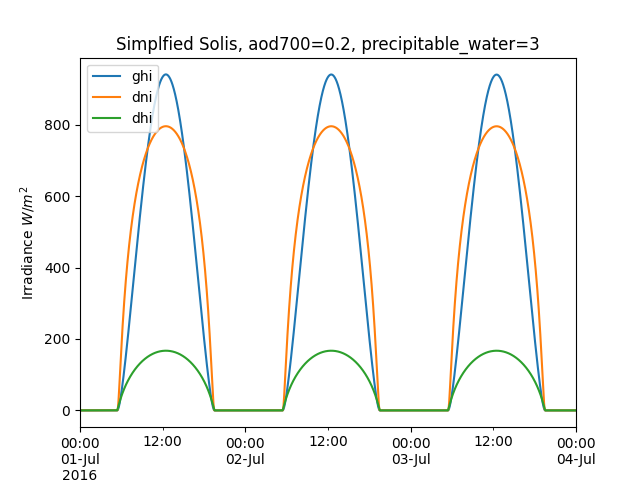See the sections below for more detail on the clear sky models.

## Ineichen and Perez¶

The Ineichen and Perez clear sky model parameterizes irradiance in terms of the Linke turbidity [Ine02]. pvlib-python implements this model in the pvlib.clearsky.ineichen() function.

### Turbidity data¶

pvlib includes a file with monthly climatological turbidity values for the globe. The code below creates turbidity maps for a few months of the year. You could run it in a loop to create plots for all months.

In : import calendar

In : import os

In : import tables

In : pvlib_path = os.path.dirname(os.path.abspath(pvlib.clearsky.__file__))

In : filepath = os.path.join(pvlib_path, 'data', 'LinkeTurbidities.h5')

In : def plot_turbidity_map(month, vmin=1, vmax=100):
....:     plt.figure();
....:     with tables.open_file(filepath) as lt_h5_file:
....:         ltdata = lt_h5_file.root.LinkeTurbidity[:, :, month-1]
....:     plt.imshow(ltdata, vmin=vmin, vmax=vmax);
....:     # data is in units of 20 x turbidity
....:     plt.title('Linke turbidity x 20, ' + calendar.month_name[month]);
....:     plt.colorbar(shrink=0.5);
....:     plt.tight_layout();
....:

In : plot_turbidity_map(1)

In : plot_turbidity_map(7)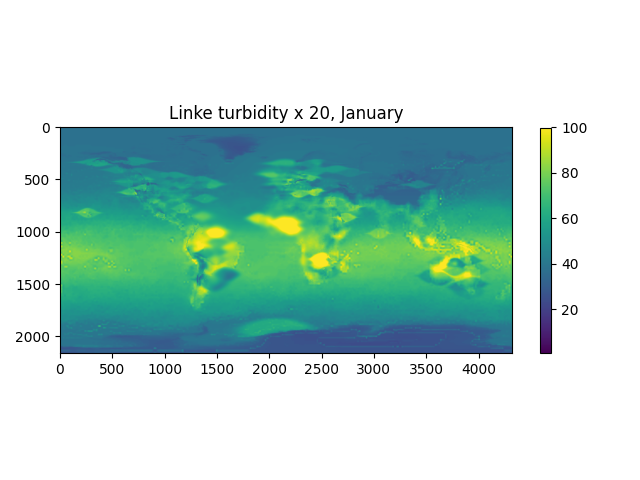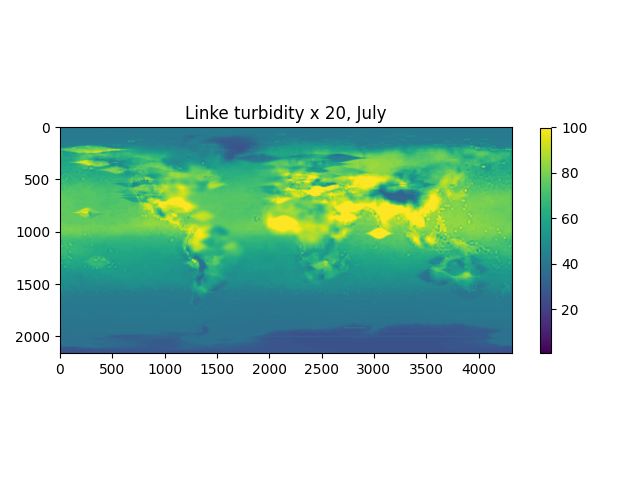The lookup_linke_turbidity() function takes a time, latitude, and longitude and gets the corresponding climatological turbidity value for that time at those coordinates. By default, the lookup_linke_turbidity() function will linearly interpolate turbidity from month to month, assuming that the raw data is valid on 15th of each month. This interpolation removes discontinuities in multi-month PV models. Here’s a plot of a few locations in the Southwest U.S. with and without interpolation. We chose points that are relatively close so that you can get a better sense of the spatial noise and variability of the data set. Note that the altitude of these sites varies from 300 m to 1500 m.

In : times = pd.date_range(start='2015-01-01', end='2016-01-01', freq='1D')

In : sites = [(32, -111, 'Tucson1'), (32.2, -110.9, 'Tucson2'),
....:          (33.5, -112.1, 'Phoenix'), (35.1, -106.6, 'Albuquerque')]
....:

In : plt.figure();

In : for lat, lon, name in sites:
....:     turbidity = pvlib.clearsky.lookup_linke_turbidity(times, lat, lon, interp_turbidity=False)
....:     turbidity.plot(label=name)
....:

In : plt.legend();

In : plt.title('Raw data (no interpolation)');

In : plt.figure();

In : for lat, lon, name in sites:
....:     turbidity = pvlib.clearsky.lookup_linke_turbidity(times, lat, lon)
....:     turbidity.plot(label=name)
....:

In : plt.legend();

In : plt.title('Interpolated to the day');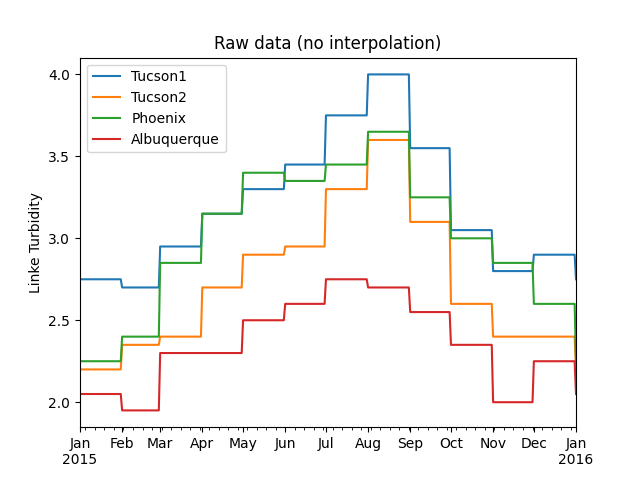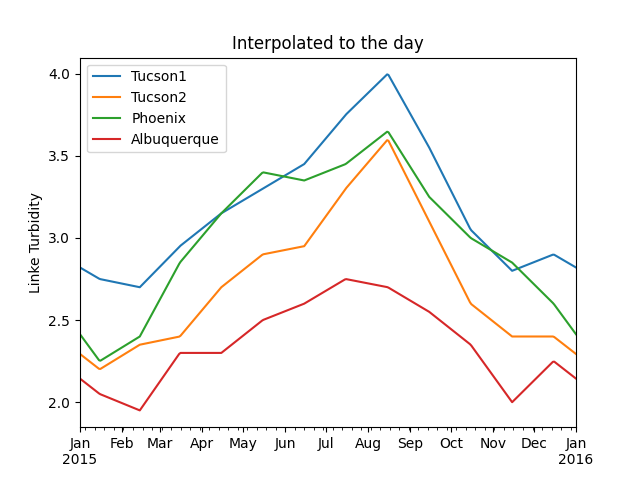The kasten96_lt() function can be used to calculate Linke turbidity [Kas96] as input to the clear sky Ineichen and Perez function. The Kasten formulation requires precipitable water and broadband aerosol optical depth (AOD). According to Molineaux, broadband AOD can be approximated by a single measurement at 700-nm [Mol98]. An alternate broadband AOD approximation from Bird and Hulstrom combines AOD measured at two wavelengths [Bir80], and is implemented in bird_hulstrom80_aod_bb().

In : pvlib_data = os.path.join(os.path.dirname(pvlib.__file__), 'data')

In : mbars = 100  # conversion factor from mbars to Pa

In : tmy_file = os.path.join(pvlib_data, '703165TY.csv')  # TMY file

....:

In : solpos = solarposition.get_solarposition(time=tmy_data.index,
....:     temperature=tmy_data['DryBulb'])
....:

In : am_rel = atmosphere.get_relative_airmass(solpos.apparent_zenith)

In : am_abs = atmosphere.get_absolute_airmass(am_rel, tmy_data['Pressure']*mbars)

In : airmass = pd.concat([am_rel, am_abs], axis=1).rename(
....:     columns={0: 'airmass_relative', 1: 'airmass_absolute'})
....:

In : tl_calculated = atmosphere.kasten96_lt(
....:     airmass.airmass_absolute, tmy_data['Pwat'], tmy_data['AOD'])
....:

In : tl = pd.concat([tl_historic, tl_calculated], axis=1).rename(
....:     columns={0:'Historic', 1:'Calculated'})
....:

In : tl.index = tmy_data.index.tz_convert(None)  # remove timezone

In : tl.resample('W').mean().plot();

In : plt.grid()

In : plt.title('Comparison of Historic Linke Turbidity Factors vs. \n'
....:     'Kasten Pyrheliometric Formula at {name:s}, {state:s} ({usaf:d}TY)'.format(
....:

In : plt.ylabel('Linke Turbidity Factor, TL');

In : plt.tight_layout()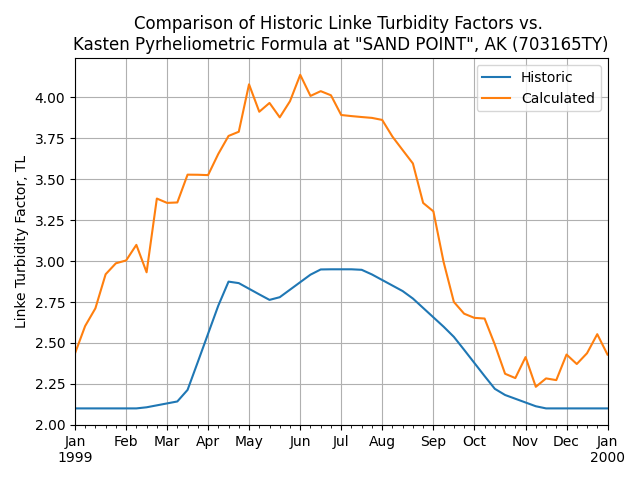### Examples¶

A clear sky time series using only basic pvlib functions.

In : latitude, longitude, tz, altitude, name = 32.2, -111, 'US/Arizona', 700, 'Tucson'

In : times = pd.date_range(start='2014-01-01', end='2014-01-02', freq='1Min', tz=tz)

In : solpos = pvlib.solarposition.get_solarposition(times, latitude, longitude)

In : apparent_zenith = solpos['apparent_zenith']

In : airmass = pvlib.atmosphere.get_relative_airmass(apparent_zenith)

In : pressure = pvlib.atmosphere.alt2pres(altitude)

In : airmass = pvlib.atmosphere.get_absolute_airmass(airmass, pressure)

# an input is a pandas Series, so solis is a DataFrame
In : ineichen = clearsky.ineichen(apparent_zenith, airmass, linke_turbidity, altitude, dni_extra)

In : plt.figure();

In : ax = ineichen.plot()

In : ax.set_ylabel('Irradiance $W/m^2$');

In : ax.set_title('Ineichen Clear Sky Model');

In : ax.legend(loc=2);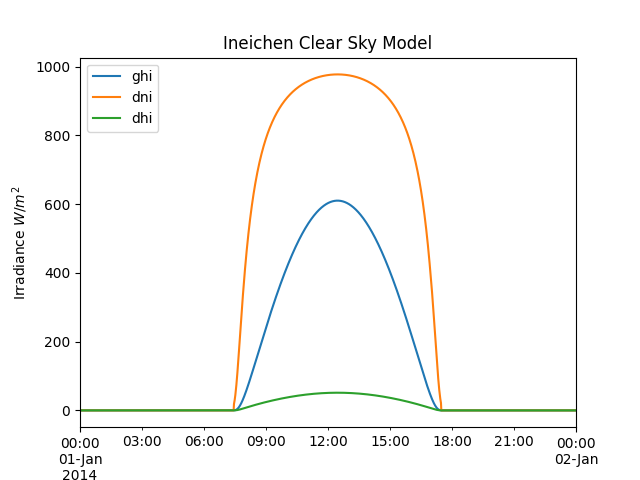The input data types determine the returned output type. Array input results in an OrderedDict of array output, and Series input results in a DataFrame output. The keys are ‘ghi’, ‘dni’, and ‘dhi’.

Grid with a clear sky irradiance for a few turbidity values.

In : times = pd.date_range(start='2014-09-01', end='2014-09-02', freq='1Min', tz=tz)

In : solpos = pvlib.solarposition.get_solarposition(times, latitude, longitude)

In : apparent_zenith = solpos['apparent_zenith']

In : airmass = pvlib.atmosphere.get_relative_airmass(apparent_zenith)

In : pressure = pvlib.atmosphere.alt2pres(altitude)

In : airmass = pvlib.atmosphere.get_absolute_airmass(airmass, pressure)

In : fig, axes = plt.subplots(ncols=3, nrows=1, sharex=True, sharey=True, squeeze=True, figsize=(12, 4))

In : axes = axes.flatten()

....:     ineichen = clearsky.ineichen(apparent_zenith, airmass, linke_turbidity, altitude, dni_extra)
....:

In : ax.legend(loc=1);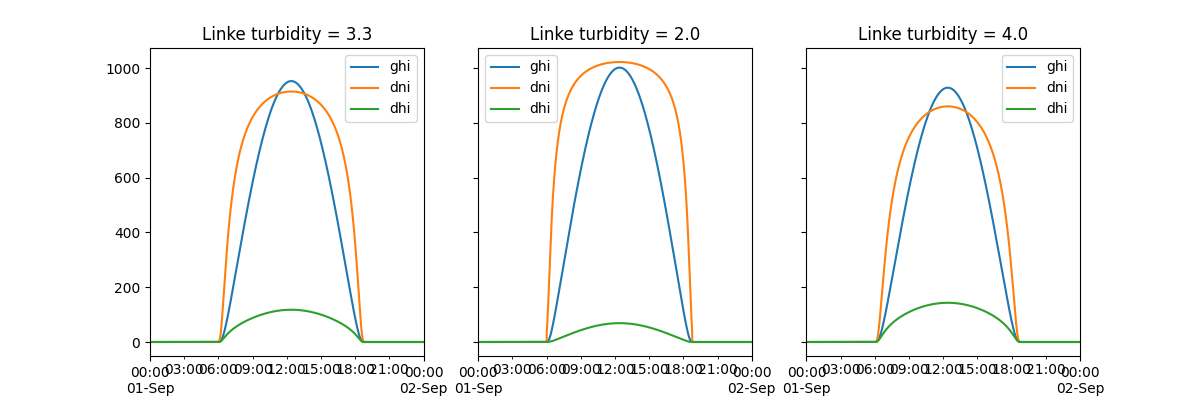### Validation¶

See [Ine02], [Ren12].

Will Holmgren compared pvlib’s Ineichen model and climatological turbidity to SoDa’s McClear service in Arizona. Here are links to an ipynb notebook and its html rendering.

## Simplified Solis¶

The Simplified Solis model parameterizes irradiance in terms of precipitable water and aerosol optical depth [Ine08ss]. pvlib-python implements this model in the pvlib.clearsky.simplified_solis() function.

### Aerosol and precipitable water data¶

There are a number of sources for aerosol and precipitable water data of varying accuracy, global coverage, and temporal resolution. Ground based aerosol data can be obtained from Aeronet. Precipitable water can be obtained from radiosondes, ESRL GPS-MET, or derived from surface relative humidity using functions such as pvlib.atmosphere.gueymard94_pw(). Numerous gridded products from satellites, weather models, and climate models contain one or both of aerosols and precipitable water. Consider data from the ECMWF and SoDa.

Aerosol optical depth (AOD) is a function of wavelength, and the Simplified Solis model requires AOD at 700 nm. angstrom_aod_at_lambda() is useful for converting AOD between different wavelengths using the Angstrom turbidity model. The Angstrom exponent, $$\alpha$$, can be calculated from AOD at two wavelengths with angstrom_alpha(). [Ine08con], [Ine16], [Ang61].

In : aod1240nm = 1.2  # fictitious AOD measured at 1240-nm

In : aod550nm = 3.1  # fictitious AOD measured at 550-nm

In : alpha_exponent = atmosphere.angstrom_alpha(aod1240nm, 1240, aod550nm, 550)

In : aod700nm = atmosphere.angstrom_aod_at_lambda(aod1240nm, 1240, alpha_exponent, 700)

In : aod380nm = atmosphere.angstrom_aod_at_lambda(aod550nm, 550, alpha_exponent, 380)

In : aod500nm = atmosphere.angstrom_aod_at_lambda(aod550nm, 550, alpha_exponent, 500)

In : aod_bb = atmosphere.bird_hulstrom80_aod_bb(aod380nm, aod500nm)

In : print('compare AOD at 700-nm = {:g}, to estimated broadband AOD = {:g}, '
....:     'with alpha = {:g}'.format(aod700nm, aod_bb, alpha_exponent))
....:
compare AOD at 700-nm = 2.33931, to estimated broadband AOD = 2.52936, with alpha = 1.16745


### Examples¶

A clear sky time series using only basic pvlib functions.

In : latitude, longitude, tz, altitude, name = 32.2, -111, 'US/Arizona', 700, 'Tucson'

In : times = pd.date_range(start='2014-01-01', end='2014-01-02', freq='1Min', tz=tz)

In : solpos = pvlib.solarposition.get_solarposition(times, latitude, longitude)

In : apparent_elevation = solpos['apparent_elevation']

In : aod700 = 0.1

In : precipitable_water = 1

In : pressure = pvlib.atmosphere.alt2pres(altitude)

# an input is a Series, so solis is a DataFrame
In : solis = clearsky.simplified_solis(apparent_elevation, aod700, precipitable_water,
.....:                                   pressure, dni_extra)
.....:

In : ax = solis.plot();

In : ax.set_ylabel('Irradiance $W/m^2$');

In : ax.set_title('Simplified Solis Clear Sky Model');

In : ax.legend(loc=2);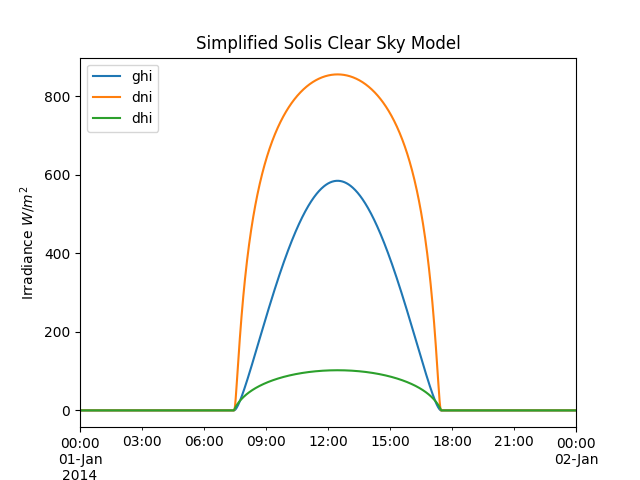The input data types determine the returned output type. Array input results in an OrderedDict of array output, and Series input results in a DataFrame output. The keys are ‘ghi’, ‘dni’, and ‘dhi’.

Irradiance as a function of solar elevation.

In : apparent_elevation = pd.Series(np.linspace(-10, 90, 101))

In : aod700 = 0.1

In : precipitable_water = 1

In : pressure = 101325

In : dni_extra = 1364

In : solis = clearsky.simplified_solis(apparent_elevation, aod700,
.....:                                   precipitable_water, pressure, dni_extra)
.....:

In : ax = solis.plot();

In : ax.set_xlabel('Apparent elevation (deg)');

In : ax.set_ylabel('Irradiance $W/m^2$');

In : ax.set_title('Irradiance vs Solar Elevation')
Out: Text(0.5, 1.0, 'Irradiance vs Solar Elevation')

In : ax.legend(loc=2);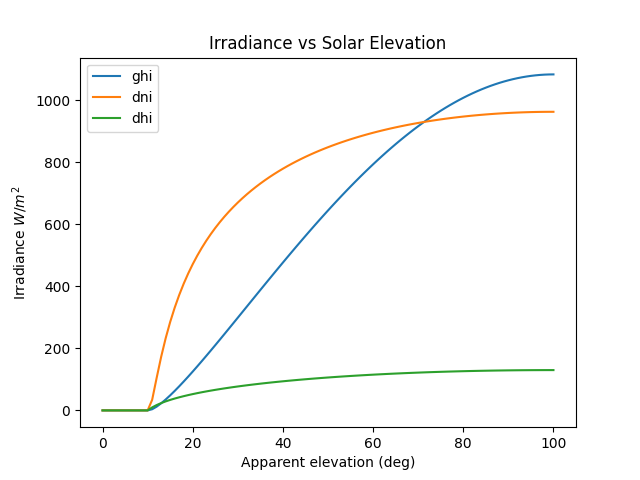Grid with clear sky irradiance for a few PW and AOD values.

In : times = pd.date_range(start='2014-09-01', end='2014-09-02', freq='1Min', tz=tz)

In : solpos = pvlib.solarposition.get_solarposition(times, latitude, longitude)

In : apparent_elevation = solpos['apparent_elevation']

In : pressure = pvlib.atmosphere.alt2pres(altitude)

In : aod700 = [0.01, 0.1]

In : precipitable_water = [0.5, 5]

In : fig, axes = plt.subplots(ncols=2, nrows=2, sharex=True, sharey=True, squeeze=True)

In : axes = axes.flatten()

In : for (aod, pw), ax in zip(itertools.chain(itertools.product(aod700, precipitable_water)), axes):
.....:     cs = clearsky.simplified_solis(apparent_elevation, aod, pw, pressure, dni_extra)
.....:     cs.plot(ax=ax, title='aod700={}, pw={}'.format(aod, pw))
.....: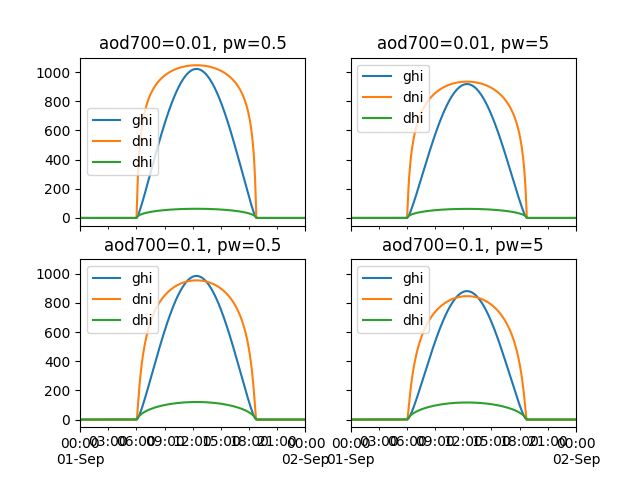Contour plots of irradiance as a function of both PW and AOD.

In : aod700 = np.linspace(0, 0.5, 101)

In : precipitable_water = np.linspace(0, 10, 101)

In : apparent_elevation = 70

In : pressure = 101325

In : dni_extra = 1364

In : aod700, precipitable_water = np.meshgrid(aod700, precipitable_water)

# inputs are arrays, so solis is an OrderedDict
In : solis = clearsky.simplified_solis(apparent_elevation, aod700,
.....:                                   precipitable_water, pressure,
.....:                                   dni_extra)
.....:

In : n = 15

In : vmin = None

In : vmax = None

In : def plot_solis(key):
.....:     fig, ax = plt.subplots()
.....:     im = ax.contour(aod700, precipitable_water, irrad[:, :], n, vmin=vmin, vmax=vmax)
.....:     imf = ax.contourf(aod700, precipitable_water, irrad[:, :], n, vmin=vmin, vmax=vmax)
.....:     ax.set_xlabel('AOD')
.....:     ax.set_ylabel('Precipitable water (cm)')
.....:     ax.clabel(im, colors='k', fmt='%.0f')
.....:     fig.colorbar(imf, label='{} (W/m**2)'.format(key))
.....:     ax.set_title('{}, elevation={}'.format(key, apparent_elevation))
.....:

In : plot_solis('ghi')

In : plot_solis('dni')

In : plot_solis('dhi')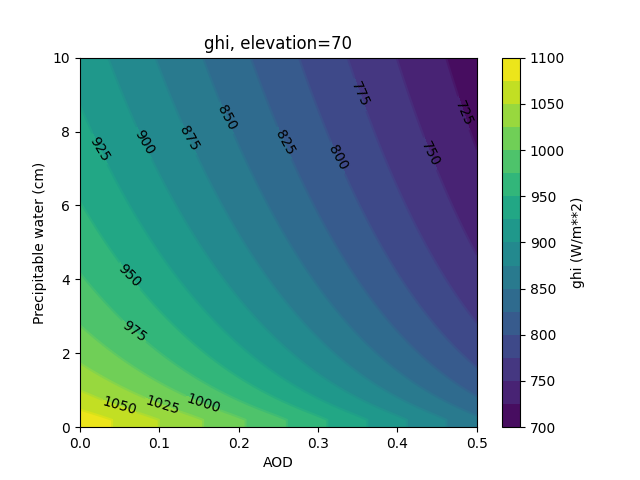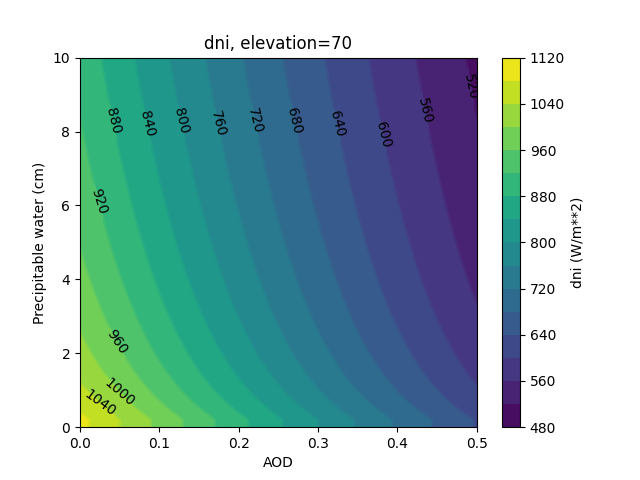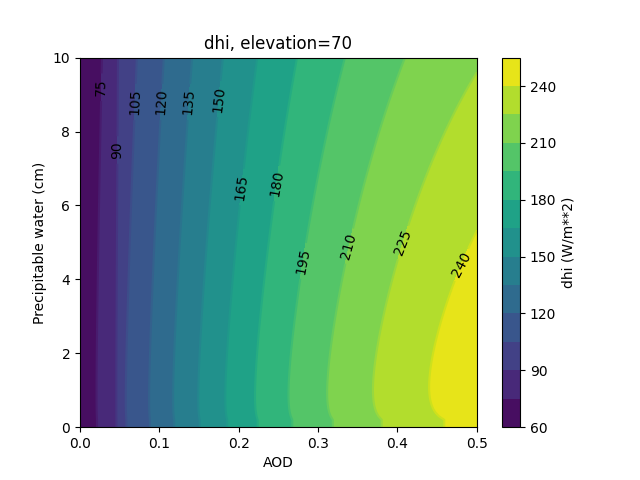### Validation¶

See [Ine16].

We encourage users to compare the pvlib implementation to Ineichen’s Excel tool.

## Detect Clearsky¶

The detect_clearsky() function implements the [Ren16] algorithm to detect the clear and cloudy points of a time series. The algorithm was designed and validated for analyzing GHI time series only. Users may attempt to apply it to other types of time series data using different filter settings, but should be skeptical of the results.

The algorithm detects clear sky times by comparing statistics for a measured time series and an expected clearsky time series. Statistics are calculated using a sliding time window (e.g., 10 minutes). An iterative algorithm identifies clear periods, uses the identified periods to estimate bias in the clearsky data, scales the clearsky data and repeats.

Clear times are identified by meeting 5 criteria. Default values for these thresholds are appropriate for 10 minute windows of 1 minute GHI data.

Next, we show a simple example of applying the algorithm to synthetic GHI data. We first generate and plot the clear sky and measured data.

In : abq = Location(35.04, -106.62, altitude=1619)

In : times = pd.date_range(start='2012-04-01 10:30:00', tz='Etc/GMT+7', periods=30, freq='1min')

In : cs = abq.get_clearsky(times)

# scale clear sky data to account for possibility of different turbidity
In : ghi = cs['ghi']*.953

In : ghi['2012-04-01 10:42:00':'2012-04-01 10:44:00'] = [500, 300, 400]

In : ghi['2012-04-01 10:56:00'] = 950

In : fig, ax = plt.subplots()

In : ghi.plot(label='input');

In : cs['ghi'].plot(label='ineichen clear');

In : ax.set_ylabel('Irradiance $W/m^2$');

In : plt.legend(loc=4);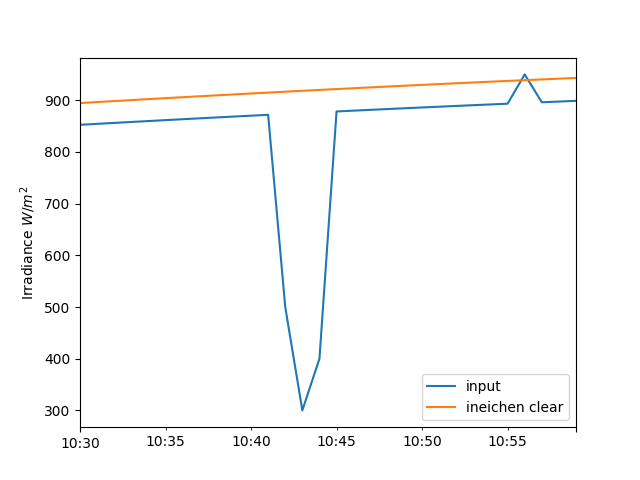Now we run the synthetic data and clear sky estimate through the detect_clearsky() function.

In : clear_samples = clearsky.detect_clearsky(ghi, cs['ghi'], cs.index, 10)

In : fig, ax = plt.subplots()

In : clear_samples.astype(int).plot();

In : ax.set_ylabel('Clear (1) or Cloudy (0)');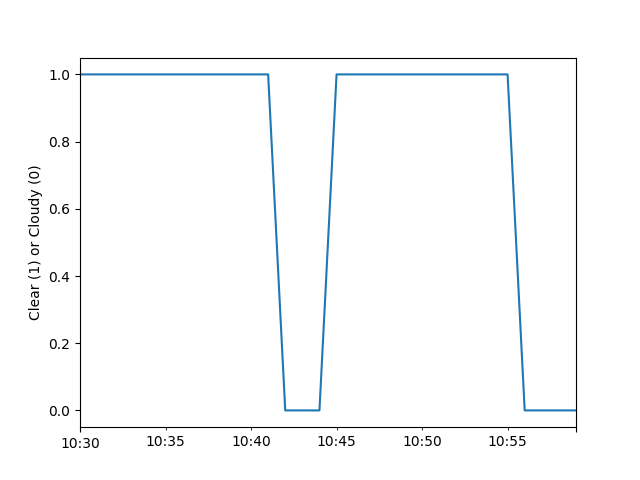The algorithm detected the cloud event and the overirradiance event.